# Excel VBA Range Tutorial

## The VBA Range Object

The Excel Range Object is an object in Excel VBA that represents a cell, row, column, a selection of cells or a 3 dimensional range. The Excel Range is also a Worksheet property that returns a subset of its cells.

Contents

## Worksheet Range

The Range is a Worksheet property which allows you to select any subset of cells, rows, columns etc.

```Dim r as Range 'Declared Range variable

Set r = Range("A1") 'Range of A1 cell

Set r = Range("A1:B2") 'Square Range of 4 cells - A1,A2,B1,B2

Set r= Range(Range("A1"), Range ("B1")) 'Range of 2 cells A1 and B1

Range("A1:B2").Select 'Select the Cells A1:B2 in your Excel Worksheet

Range("A1:B2").Activate 'Activate the cells and show them on your screen (will switch to Worksheet and/or scroll to this range.
```

Select a cell or Range of cells using the Select method. It will be visibly marked in Excel:### Working with Range variables

The Range is a separate object variable and can be declared as other variables. As the VBA Range is an object you need to use the Set statement:

```Dim myRange as Range
'...
Set myRange = Range("A1") 'Need to use Set to define myRange
```

The Range object defaults to your ActiveWorksheet. So beware as depending on your ActiveWorksheet the Range object will return values local to your worksheet:

```Range("A1").Select
'...is the same as...
ActiveSheet.Range("A1").Select
```

You might want to define the Worksheet reference by Range if you want your reference values from a specifc Worksheet:

```Sheets("Sheet1").Range("A1").Select 'Will always select items from Worksheet named Sheet1
```
The ActiveWorkbook is not same to ThisWorkbook. Same goes for the ActiveSheet. This may reference a Worksheet from within a Workbook external to the Workbook in which the macro is executed as Active references simply the currently top-most worksheet. Read more here

### Range properties

The Range object contains a variety of properties with the main one being it’s Value and an the second one being its Formula.

A Range Value is the evaluated property of a cell or a range of cells. For example a cell with the formula `=10+20` has an evaluated value of `20`.
A Range Formula is the formula provided in the cell or range of cells. For example a cell with a formula of `=10+20` will have the same Formula property.

```'Let us assume A1 contains the formula "=10+20"

Debug.Print Range("A1").Value 'Returns: 30

Debug.Print Range("A1").Formula 'Returns: =10+20```

Other Range properties include:
Work in progress

## Worksheet Cells

A Worksheet Cells property is similar to the Range property but allows you to obtain only a SINGLE CELL, based on its row and column index. Numbering starts at 1:The Cells property is in fact a Range object not a separate data type.
Excel facilitates a Cells function that allows you to obtain a cell from within the ActiveSheet, current top-most worksheet.

```Cells(2,2).Select 'Selects B2
'...is the same as...
ActiveSheet.Cells(2,2).Select 'Select B2
```

Cells are Ranges which means they are not a separate data type:

```Dim myRange as Range
Set myRange = Cells(1,1) 'Cell A1
```

## Range Rows and Columns

As we all know an Excel Worksheet is divided into Rows and Columns. The Excel VBA Range object allows you to select single or multiple rows as well as single or multiple columns. There are a couple of ways to obtain Worksheet rows in VBA:

### Getting an entire row or column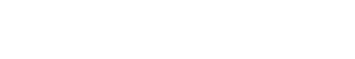To get and entire row of a specified Range you need to use the EntireRow property. Although, the function’s parameters suggest taking both a RowIndex and ColumnIndex it is enough just to provide the row number. Row indexing starts at 1.To get and entire column of a specified Range you need to use the EntireColumn property. Although, the function’s parameters suggest taking both a RowIndex and ColumnIndex it is enough just to provide the column number. Column indexing starts at 1.

```Range("B2").EntireRows(1).Hidden = True 'Gets and hides the entire row 2

Range("B2").EntireColumns(1).Hidden = True 'Gets and hides the entire column 2
```
The three properties EntireRow/EntireColumn, Rows/Columns and Row/Column are often misunderstood so read through to understand the differences.

### Get a row/column of a specified rangeIf you want to get a certain row within a Range simply use the Rows property of the Worksheet. Although, the function’s parameters suggest taking both a RowIndex and ColumnIndex it is enough just to provide the row number. Row indexing starts at 1.Similarly you can use the Columns function to obtain any single column within a Range. Although, the function’s parameters suggest taking both a RowIndex and ColumnIndex actually the first argument you provide will be the column index. Column indexing starts at 1.

```Rows(1).Hidden = True 'Hides the first row in the ActiveSheet
'same as
ActiveSheet.Rows(1).Hidden = True

Columns(1).Hidden = True 'Hides the first column in the ActiveSheet
'same as
ActiveSheet.Columns(1).Hidden = True
```

To get a range of rows/columns you need to use the Range function like so:

```Range(Rows(1), Rows(3)).Hidden = True 'Hides rows 1:3
'same as
Range("1:3").Hidden = True
'same as
ActiveSheet.Range("1:3").Hidden = True

Range(Columns(1), Columns(3)).Hidden = True 'Hides columns A:C
'same as
Range("A:C").Hidden = True
'same as
ActiveSheet.Range("A:C").Hidden = True
```

### Get row/column of specified range

The above approach assumed you want to obtain only rows/columns from the ActiveSheet – the visible and top-most Worksheet. Usually however, you will want to obtain rows or columns of an existing Range. Similarly as with the Worksheet Range property, any Range facilitates the Rows and Columns property.

```Dim myRange as Range
Set myRange = Range("A1:C3")

myRange.Rows.Hidden = True 'Hides rows 1:3
myRange.Columns.Hidden = True 'Hides columns A:C

Set myRange = Range("C10:F20")
myRange.Rows(2).Hidden = True 'Hides rows 11
myRange.Columns(3).Hidden = True 'Hides columns E
```

#### Getting a Ranges first row/column number

Aside from the Rows and Columns properties Ranges also facilitate a Row and Column property which provide you with the number of the Ranges first row and column.

```Set myRange = Range("C10:F20")

'Get first row number
Debug.Print myRange.Row 'Result: 10
'Get first column number
Debug.Print myRange.Column 'Result: 3
```

#### Converting Column number to Excel Column

This is an often question that turns up – how to convert a column number to a string e.g. 100 to “CV”.

```Function GetExcelColumn(columnNumber As Long)
Dim div As Long, colName As String, modulo As Long
div = columnNumber: colName = vbNullString

Do While div > 0
modulo = (div - 1) Mod 26
colName = Chr(65 + modulo) & colName
div = ((div - modulo) / 26)
Loop

GetExcelColumn = colName
End Function
```

## Range Cut/Copy/Paste

Cutting and pasting rows is generally a bad practice which I heavily discourage as this is a practice that is moments can be heavily cpu-intensive and often is unaccounted for.

### Copy functionThe Copy function works on a single cell, subset of cell or subset of rows/columns.

```'Copy values and formatting from cell A1 to cell D1
Range("A1").Copy Range("D1")

'Copy 3x3 A1:C3 matrix to D1:F3 matrix - dimension must be same
Range("A1:C3").Copy Range("D1:F3")

'Copy rows 1:3 to rows 4:6
Range("A1:A3").EntireRow.Copy Range("A4")

'Copy columns A:C to columns D:F
Range("A1:C1").EntireColumn.Copy Range("D1")
```

The Copy function can also be executed without an argument. It then copies the Range to the Windows Clipboard for later Pasting.

### Cut function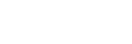The Cut function, similarly as the Copy function, cuts single cells, ranges of cells or rows/columns.

```'Cut A1 cell and paste it to D1
Range("A1").Cut Range("D1")

'Cut 3x3 A1:C3 matrix and paste it in D1:F3 matrix - dimension must be same
Range("A1:C3").Cut Range("D1:F3")

'Cut rows 1:3 and paste to rows 4:6
Range("A1:A3").EntireRow.Cut Range("A4")

'Cut columns A:C and paste to columns D:F
Range("A1:C1").EntireColumn.Cut Range("D1")
```

The Cut function can be executed without arguments. It will then cut the contents of the Range and copy it to the Windows Clipboard for pasting.

Cutting cells/rows/columns does not shift any remaining cells/rows/columns but simply leaves the cut out cells empty

### PasteSpecial functionThe Range PasteSpecial function works only when preceded with either the Copy or Cut Range functions. It pastes the Range (or other data) within the Clipboard to the Range on which it was executed.

#### Syntax

The PasteSpecial function has the following syntax:

```PasteSpecial( Paste, Operation, SkipBlanks, Transpose)
```
The PasteSpecial function can only be used in tandem with the Copy function (not Cut)
##### Parameters

Paste
The part of the Range which is to be pasted. This parameter can have the following values:

Parameter Constant Description
xlPasteSpecialOperationAdd 2 Copied data will be added with the value in the destination cell.
xlPasteSpecialOperationDivide 5 Copied data will be divided with the value in the destination cell.
xlPasteSpecialOperationMultiply 4 Copied data will be multiplied with the value in the destination cell.
xlPasteSpecialOperationNone -4142 No calculation will be done in the paste operation.
xlPasteSpecialOperationSubtract 3 Copied data will be subtracted with the value in the destination cell.

Operation
The paste operation e.g. paste all, only formatting, only values, etc. This can have one of the following values:

Name Constant Description
xlPasteAll -4104 Everything will be pasted.
xlPasteAllExceptBorders 7 Everything except borders will be pasted.
xlPasteAllMergingConditionalFormats 14 Everything will be pasted and conditional formats will be merged.
xlPasteAllUsingSourceTheme 13 Everything will be pasted using the source theme.
xlPasteColumnWidths 8 Copied column width is pasted.
xlPasteFormats -4122 Copied source format is pasted.
xlPasteFormulas -4123 Formulas are pasted.
xlPasteFormulasAndNumberFormats 11 Formulas and Number formats are pasted.
xlPasteValidation 6 Validations are pasted.
xlPasteValues -4163 Values are pasted.
xlPasteValuesAndNumberFormats 12 Values and Number formats are pasted.

SkipBlanks
If True then blanks will not be pasted.

Transpose
Transpose the Range before paste (swap rows with columns).

#### PasteSpecial Examples

```'Cut A1 cell and paste its values to D1
Range("A1").Copy
Range("D1").PasteSpecial

'Copy 3x3 A1:C3 matrix and add all the values to E1:G3 matrix (dimension must be same)
Range("A1:C3").Copy
```

Below an example where the Excel Range A1:C3 values are copied an added to the E1:G3 Range. You can also multiply, divide and run other similar operations.### Paste

The Paste function allows you to paste data in the Clipboard to the actively selected Range. Cutting and Pasting can only be accomplished with the Paste function.

```'Cut A1 cell and paste its values to D1
Range("A1").Cut
Range("D1").Select
ActiveSheet.Paste

'Cut 3x3 A1:C3 matrix and paste it in D1:F3 matrix - dimension must be same
Range("A1:C3").Cut
Range("D1:F3").Select
ActiveSheet.Paste

'Cut rows 1:3 and paste to rows 4:6
Range("A1:A3").EntireRow.Cut
Range("A4").Select
ActiveSheet.Paste

'Cut columns A:C and paste to columns D:F
Range("A1:C1").EntireColumn.Cut
Range("D1").Select
ActiveSheet.Paste
```

## Range Clear/Delete

### The Clear function

The Clear function clears the entire content and formatting from an Excel Range. It does not, however, shift (delete) the cleared cells.

```Range("A1:C3").Clear
```### The Delete function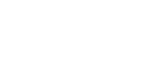The Delete function deletes a Range of cells, removing them entirely from the Worksheet, and shifts the remaining Cells in a selected shift direction.
Although the manual Delete cell function provides 4 ways of shifting cells. The VBA Delete Shift values can only be either be xlShiftToLeft or xlShiftUp.

```'If Shift omitted, Excel decides - shift up in this case
Range("B2").Delete

'Delete and Shift remaining cells left
Range("B2").Delete xlShiftToLeft

'Delete and Shift remaining cells up
Range("B2").Delete xlShiftTop

'Delete entire row 2 and shift up
Range("B2").EntireRow.Delete

'Delete entire column B and shift left
Range("B2").EntireRow.Delete
```## Traversing Ranges

Traversing cells is really useful when you want to run an operation on each cell within an Excel Range. Fortunately this is easily achieved in VBA using the For Each or For loops.

```Dim cellRange As Range

For Each cellRange In Range("A1:C3")
Debug.Print cellRange.Value
Next cellRange
```
Although this may not be obvious, beware of iterating/traversing the Excel Range using a simple For loop. For loops are not efficient on Ranges. Use a For Each loop as shown above. This is because Ranges resemble more Collections than Arrays. Read more on For vs For Each loops here

### Traversing the UsedRangeEvery Worksheet has a UsedRange. This represents that smallest rectangle Range that contains all cells that have or had at some point values. In other words if the further out in the bottom, right-corner of the Worksheet there is a certain cell (e.g. E8) then the UsedRange will be as large as to include that cell starting at cell A1 (e.g. A1:E8). In Excel you can check the current UsedRange hitting CTRL+END. In VBA you get the UsedRange like this:

```ActiveSheet.UsedRange
'same as
UsedRange
```

You can traverse through the UsedRange like this:

```Dim cellRange As Range

For Each cellRange In UsedRange
Debug.Print "Row: " & cellRange.Row & ", Column: " & cellRange.Column
Next cellRange
```
The UsedRange is a useful construct responsible often for bloated Excel Workbooks. Often delete unused Rows and Columns that are considered to be within the UsedRange can result in significantly reducing your file size. Read also more on the XSLB file format here

The Excel Range Address property provides a string value representing the Address of the Range.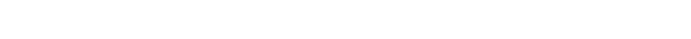### Syntax

Below the syntax of the Excel Range Address property:

```Address( [RowAbsolute], [ColumnAbsolute], [ReferenceStyle], [External], [RelativeTo] )
```

#### Parameters

RowAbsolute
Optional. If True returns the row part of the reference address as an absolute reference. By default this is True.

```\$D\$10:\$G\$100 'RowAbsolute is set to True
\$D10:\$G100 'RowAbsolute is set to False
```

ColumnAbsolute
Optional. If True returns the column part of the reference as an absolute reference. By default this is True.

```\$D\$10:\$G\$100 'ColumnAbsolute is set to True
D\$10:G\$100 'ColumnAbsolute is set to False
```

ReferenceStyle
Optional. The reference style. The default value is xlA1. Possible values:

Constant Value Description
xlA1 1 Default. Use xlA1 to return an A1-style reference
xlR1C1 -4150 Use xlR1C1 to return an R1C1-style reference

External
Optional. If True then property will return an external reference address, otherwise a local reference address will be returned. By default this is False.

```\$A\$1 'Local
[Book1.xlsb]Sheet1!\$A\$1 'External
```

RelativeTo
Provided RowAbsolute and ColumnAbsolute are set to False, and the ReferenceStyle is set to xlR1C1, then you must include a starting point for the relative reference. This must be a Range variable to be set as the reference point.

## Merged Ranges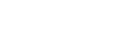Merged cells are Ranges that consist of 2 or more adjacent cells. To Merge a collection of adjacent cells run Merge function on that Range.

The Merge has only a single parameter – Across, a boolean which if True will merge cells in each row of the specified range as separate merged cells. Otherwise the whole Range will be merged. The default value is False.

### Merge examples

To merge the entire Range:

```'This will turn of any alerts warning that values may be lost

Range("B2:C3").Merge
```

This will result in the following:To merge just the rows set Across to True.

```'This will turn of any alerts warning that values may be lost

Range("B2:C3").Merge True
```
Remember that merged Ranges can only have a single value and formula. Hence, if you merge a group of cells with more than a single value/formula only the first value/formula will be set as the value/formula for your new merged Range

### Checking if Range is merged

To check if a certain Range is merged simply use the Excel Range MergeCells property:

```Range("B2:C3").Merge

Debug.Print Range("B2").MergeCells 'Result: True
```

### The MergeArea

The MergeArea is a property of an Excel Range that represent the whole merge Range associated with the current Range. Say that \$B\$2:\$C\$3 is a merged Range – each cell within that Range (e.g. B2, C3..) will have the exact same MergedArea. See example below:

```Range("B2:C3").Merge
```

## Named Ranges

Named Ranges are Ranges associated with a certain Name (string). In Excel you can find all your Named Ranges by going to Formulas->Name Manager. They are very useful when working on certain values that are used frequently through out your Workbook. Imagine that you are writing a Financial Analysis and want to use a common Discount Rate across all formulas. Just the address of the cell e.g. “A2”, won’t be self-explanatory. Why not use e.g. “DiscountRate” instead? Well you can do just that.

### Creating a Named Range

Named Ranges can be created either within the scope of a Workbook or Worksheet:

```Dim r as Range
'Within Workbook
'Within Worksheet
```

This gives you flexibility to use similar names across multiple Worksheets or use a single global name across the entire Workbook.

### Listing all Named Ranges

You can list all Named Ranges using the Name Excel data type. Names are objects that represent a single NamedRange. See an example below of listing our two newly created NamedRanges:

```Call ActiveWorkbook.Names.Add("NewName", Range("A1"))

Dim n As Name
For Each n In ActiveWorkbook.Names
Debug.Print "Name: " & n.Name & ", Address: " & _
n.RefersToRange.Address & ", Value: "; n.RefersToRange.Value
Next n

'Result:
'Name: Sheet1!NewName, Address: \$A\$1, Value:  1
'Name: NewName, Address: \$A\$1, Value:  1
```

## SpecialCells

SpecialCells are a very useful Excel Range property, that allows you to select a subset of cells/Ranges within a certain Range.

### Syntax

The SpecialCells property has the following syntax:

```SpecialCells( Type, [Value] )
```

#### Parameters

Type
The type of cells to be returned. Possible values:

Constant Value Description
xlCellTypeAllFormatConditions -4172 Cells of any format
xlCellTypeAllValidation -4174 Cells having validation criteria
xlCellTypeBlanks 4 Empty cells
xlCellTypeConstants 2 Cells containing constants
xlCellTypeFormulas -4123 Cells containing formulas
xlCellTypeLastCell 11 The last cell in the used range
xlCellTypeSameFormatConditions -4173 Cells having the same format
xlCellTypeSameValidation -4175 Cells having the same validation criteria
xlCellTypeVisible 12 All visible cells

Value
If Type is equal to xlCellTypeConstants or xlCellTypeFormulas this determines the types of cells to return e.g. with errors.

Constant Value
xlErrors 16
xlLogical 4
xlNumbers 1
xlTextValues 2

### SpecialCells examples

#### Get Excel Range with Constants

This will return only cells with constant cells within the Range C1:C3:

```For Each r In Range("A1:C3").SpecialCells(xlCellTypeConstants)
Debug.Print r.Value
Next r
```

#### Search for Excel Range with Errors

```For Each r In ActiveSheet.UsedRange.SpecialCells(xlCellTypeFormulas, xlErrors)